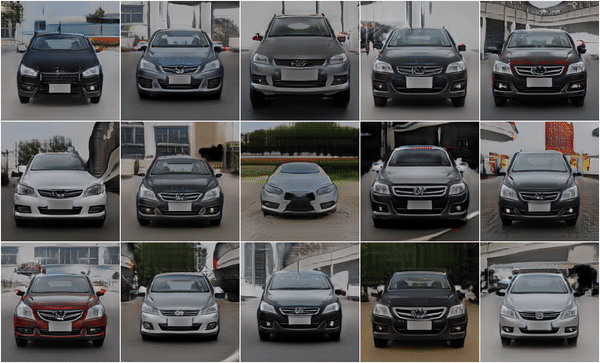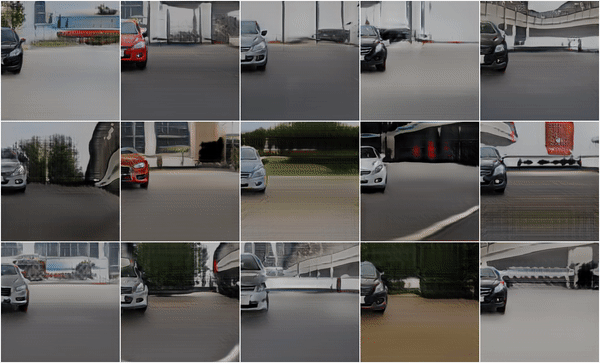# GIRAFFE： CVPR 2021 最佳论文介绍和代码解释

GIRAFFE是一个基于学习的、完全可微的渲染引擎，用于将场景合成为多个“特征域”的总和。

CVPR 2021年结束了，深度学习继续在计算机视觉领域占据主导地位，包括SLAM、姿态估计、深度估计、新的数据集、GANs，以及去年的神经辐射场或NeRFs的许多改进。

## GIRAFFE

GIRAFFE 项目包括源代码，您可以使用这些源代码来重现他们的人物，甚至创作您自己的场景。我简要介绍了他们的源代码，并展示了我们如何使用 GIRAFFE 来组成一些简单的神经 3D 场景。

GIRAFFE 源代码的结构考虑了配置。 configs/default.yaml 文件指定应用程序的默认配置。 其他配置文件，如 configs/256res/cars_256/pretrained.yaml 使用 inherit_from 键从该配置文件继承，并通过指定其他键值对覆盖默认值。 这使我们能够使用 python render.py <CONFIG.yaml> 渲染图像，并通过自记录配置文件而不是组合输入参数使用 python train.py <CONFIG.yaml> 进行训练。

``````inherit_from: configs/256res/cars_256.yaml
training:
out_dir:  out/cars256_pretrained
test:
model_file: https://s3.eu-central-1.amazonaws.com/avg-projects/giraffe/models/checkpoint_cars256-d9ea5e11.pt
rendering:
render_dir: rendering
````````````class Renderer(object):
# ...
def render_object_rotation(self, img_out_path, batch_size=15, n_steps=32):
gen = self.generator
bbox_generator = gen.bounding_box_generator

n_boxes = bbox_generator.n_boxes

# Set rotation range
is_full_rotation = (bbox_generator.rotation_range == 0
and bbox_generator.rotation_range == 1)
n_steps = int(n_steps * 2) if is_full_rotation else n_steps
r_scale = [0., 1.] if is_full_rotation else [0.1, 0.9]

# Get Random codes and bg rotation
latent_codes = gen.get_latent_codes(batch_size, tmp=self.sample_tmp)
bg_rotation = gen.get_random_bg_rotation(batch_size)

# Set Camera
camera_matrices = gen.get_camera(batch_size=batch_size)
s_val = [[0, 0, 0] for i in range(n_boxes)]
t_val = [[0.5, 0.5, 0.5] for i in range(n_boxes)]
r_val = [0. for i in range(n_boxes)]
s, t, _ = gen.get_transformations(s_val, t_val, r_val, batch_size)

out = []
for step in range(n_steps):
# Get rotation for this step
r = [step * 1.0 / (n_steps - 1) for i in range(n_boxes)]
r = [r_scale + ri * (r_scale - r_scale) for ri in r]
r = gen.get_rotation(r, batch_size)

# define full transformation and evaluate model
transformations = [s, t, r]
out_i = gen(batch_size, latent_codes, camera_matrices,
transformations, bg_rotation, mode='val')
out.append(out_i.cpu())
out = torch.stack(out)
out_folder = join(img_out_path, 'rotation_object')
makedirs(out_folder, exist_ok=True)
self.save_video_and_images(
out, out_folder, name='rotation_object',
is_full_rotation=is_full_rotation,
# ...
``````

``````from im2scene.giraffe.models import generator
# ...
generator_dict = {
'simple': generator.Generator,
}
``````

``````z_shape_obj, z_app_obj, z_shape_bg, z_app_bg = latent_codes
``````

``````class Generator(nn.Module):
# ...
def get_latent_codes(self, batch_size=32, tmp=1.):
z_dim, z_dim_bg = self.z_dim, self.z_dim_bg
n_boxes = self.get_n_boxes()
def sample_z(x): return self.sample_z(x, tmp=tmp)
z_shape_obj = sample_z((batch_size, n_boxes, z_dim))
z_app_obj = sample_z((batch_size, n_boxes, z_dim))
z_shape_bg = sample_z((batch_size, z_dim_bg))
z_app_bg = sample_z((batch_size, z_dim_bg))
return z_shape_obj, z_app_obj, z_shape_bg, z_app_bg

def sample_z(self, size, to_device=True, tmp=1.):
z = torch.randn(*size) * tmp
if to_device:
z = z.to(self.device)
return z
# ...
``````

``````n_iter = n_boxes if not_render_background else n_boxes + 1
# ...
for i in range(n_iter):
if i < n_boxes:  # Object
p_i, r_i = self.get_evaluation_points(pixels_world,
camera_world, di, transformations, i)
z_shape_i, z_app_i = z_shape_obj[:, i], z_app_obj[:, i]
feat_i, sigma_i = self.decoder(p_i, r_i, z_shape_i, z_app_i)
# ...
else:  # Background
p_bg, r_bg = self.get_evaluation_points_bg(pixels_world,
camera_world, di, bg_rotation)
feat_i, sigma_i = self.background_generator(
p_bg, r_bg, z_shape_bg, z_app_bg)
# ...
feat.append(feat_i)
sigma.append(sigma_i)
# ...
``````

``````sigma_sum, feat_weighted = self.composite_function(sigma, feat)
``````

``````weights = self.calc_volume_weights(di, ray_vector, sigma_sum)
feat_map = torch.sum(weights.unsqueeze(-1) * feat_weighted, dim=-2)
``````

``````class Renderer(object):
# ...
def render_full_visualization(self, img_out_path,
render_program=['object_rotation']):
for rp in render_program:
# ...
# APPEND THIS TO THE END OF render_full_visualization
if rp == 'object_wipeout':
self.set_random_seed()
self.render_object_wipeout(img_out_path)
# ...
# APPEND THIS TO THE END OF rendering.py
def render_object_wipeout(self, img_out_path, batch_size=15,
n_steps=32):
gen = self.generator

# Get values
latent_codes = gen.get_latent_codes(batch_size, tmp=self.sample_tmp)
bg_rotation = gen.get_random_bg_rotation(batch_size)
camera_matrices = gen.get_camera(batch_size=batch_size)
n_boxes = gen.bounding_box_generator.n_boxes
s = [[0., 0., 0.]
for i in range(n_boxes)]
n_steps = int(n_steps * 2)
r_scale = [0., 1.]

if n_boxes == 1:
t = []
x_val = 0.5
elif n_boxes == 2:
t = [[0.5, 0.5, 0.]]
x_val = 1.0

out = []
for step in range(n_steps):
# translation
i = step * 1.0 / (n_steps - 1)
ti = t + [[0.1, i, 0.]]
# rotation
r = [step * 1.0 / (n_steps - 1) for i in range(n_boxes)]
r = [r_scale + ri * (r_scale - r_scale) for ri in r]

transformations = gen.get_transformations(s, ti, r, batch_size)
out_i = gen(batch_size, latent_codes, camera_matrices,
transformations, bg_rotation, mode='val')
out.append(out_i.cpu())
out = torch.stack(out)

out_folder = join(img_out_path, 'object_wipeout')
makedirs(out_folder, exist_ok=True)
self.save_video_and_images(
out, out_folder, name='object_wipeout',
``````

``````inherit_from: configs/256res/cars_256.yaml
training:
out_dir:  out/cars256_pretrained
test:
model_file: https://s3.eu-central-1.amazonaws.com/avg-projects/giraffe/models/checkpoint_cars256-d9ea5e11.pt
rendering:
render_dir: rendering
render_program: ['object_wipeout']
``````

GIRAFFE 是对 NeRF 和 GAN 研究最近大量研究的一个令人兴奋的补充。 辐射场表示描述了一个强大且可扩展的框架，我们可以用它以可区分和可学习的方式构建 3D 场景。 我希望您发现深入了解代码很有用。 如果是这样，我鼓励您自己查看作者的来源和论文。

 Ben Mildenhall, Pratul P. Srinivasan, Matthew Tancik, Jonathan T. Barron, Ravi Ramamoorthi, Ren Ng — NeRF: Representing Scenes as Neural Radiance Fields for View Synthesis (2020), ECCV 2020

### 热门文章## jira安装配置指南（Ubuntu16.04）内含解决connection refused问题方法## 那些年让我们目瞪口呆的bug## redis的下载与安装## 《大话数据结构》第三章学习笔记--线性表（一）## 对象的扩展## 让程序员最头疼的5种编程语言## 写论文注意事项## 安卓 串口开发## 2021-2027年中国铪市场调研与发展趋势分析报告

2021-2027年中国铪市场调研与发展趋势分析报告 本报告研究中国市场铪的生产、消费及进出口情况&#xff0c;重点关注在中国市场扮演重要角色的全球及本土铪生产商&#xff0c;呈现这些厂商在中国市场的铪销量、收入、价格、毛利率、市场份额等关键指标。此外&#xff0c;针对…## Aggressive cows题目翻译## 剖析组建PMO的6个大坑︱PMO深度实践## Flowable入门系列文章118 - 进程实例 07

1、获取流程实例的变量 GET运行时/进程实例/ {processInstanceId} /变量/ {变量名} 表1.获取流程实例的变量 - URL参数 参数需要值描述processInstanceId是串将流程实例的id添加到变量中。变量名是串要获取的变量的名称。 表2.获取流程实例的变量 - 响应代码 响应码描述200指…## 微信每天自动给女[男]朋友发早安和土味情话## C语言二分查找详解## 【普通人VS程序员】电脑还可以这样关机，神操作，学到了学到了~（收藏起来慢慢学）## 项目经理，你有什么优势吗？## nginx的负载均衡和故障转移

#注&#xff1a;proxy_temp_path和proxy_cache_path指定的路径必须在同一分区 proxy_temp_path /data0/proxy_temp_dir; #设置Web缓存区名称为cache_one&#xff0c;内存缓存空间大小为200MB&#xff0c;1天没有被访问的内容自动清除&#xff0c;硬盘缓存空间大小为30GB。 pro…## 业务逻辑漏洞